# Surface Area of Prisms And Cylinders Prism Polyhedron

• Slides: 18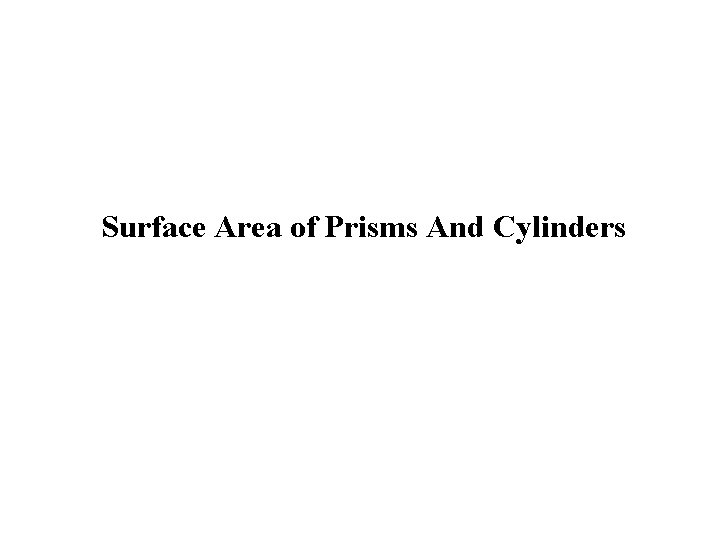Surface Area of Prisms And CylindersPrism: Polyhedron with two parallel, congruent bases Named after its baseSurface area: Sum of the area of each face of the solid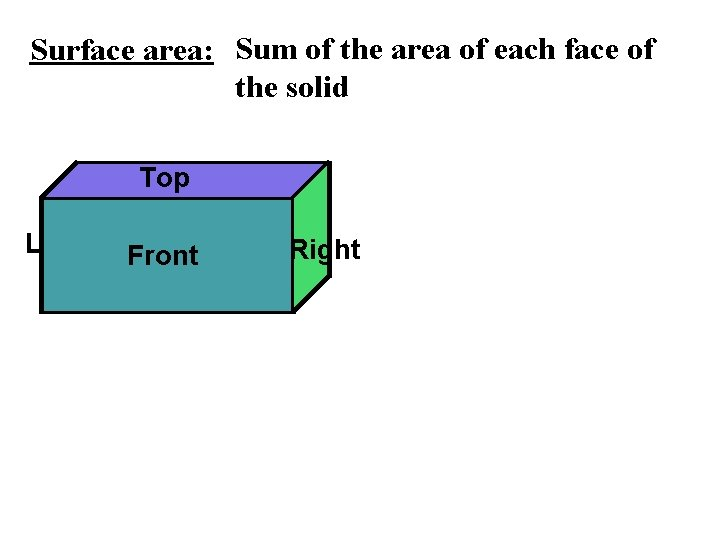Surface area: Sum of the area of each face of the solid Left Top Back Front Bottom Right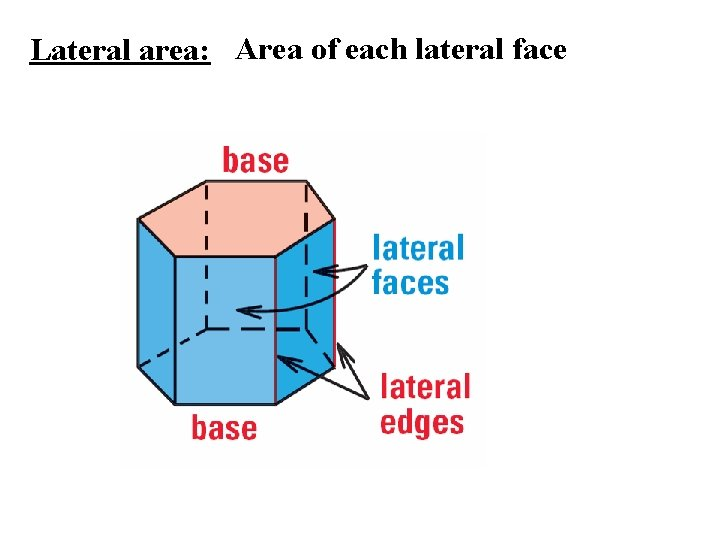Lateral area: Area of each lateral face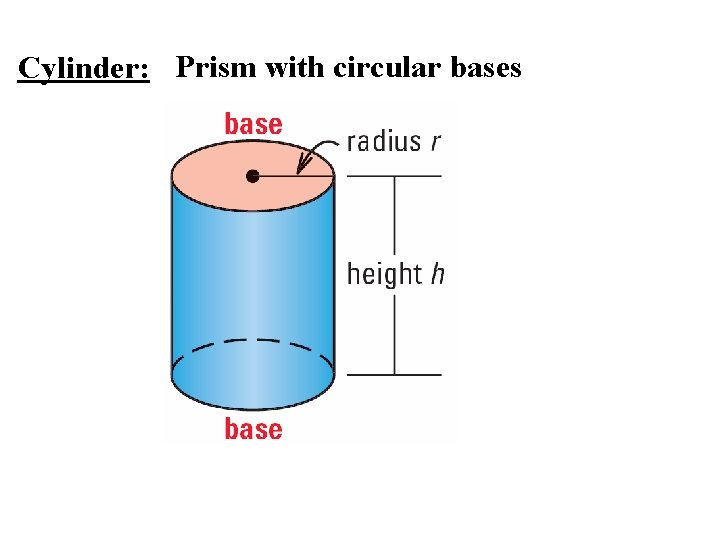Cylinder: Prism with circular bases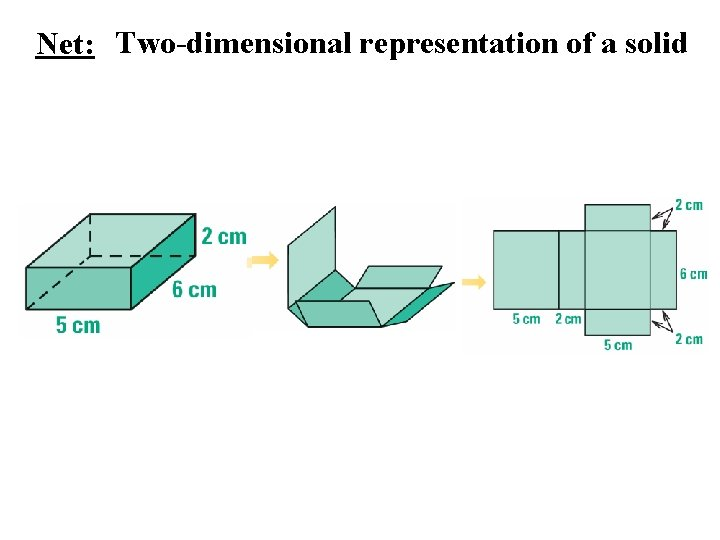Net: Two-dimensional representation of a solid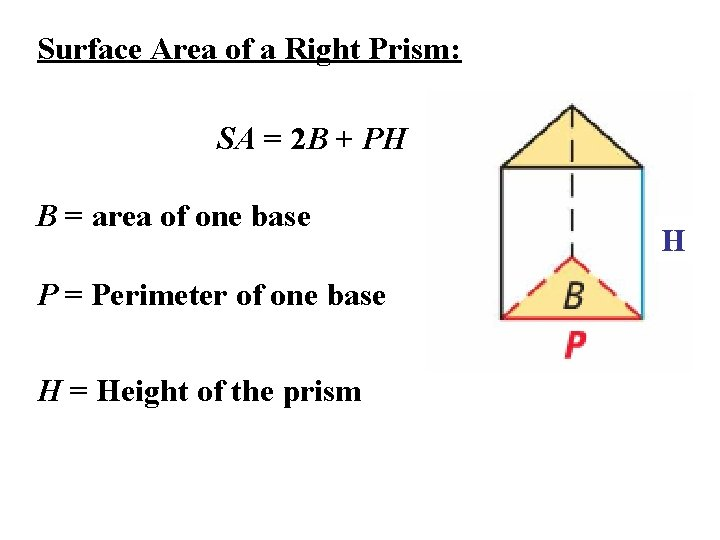Surface Area of a Right Prism: SA = 2 B + PH B = area of one base P = Perimeter of one base H = Height of the prism H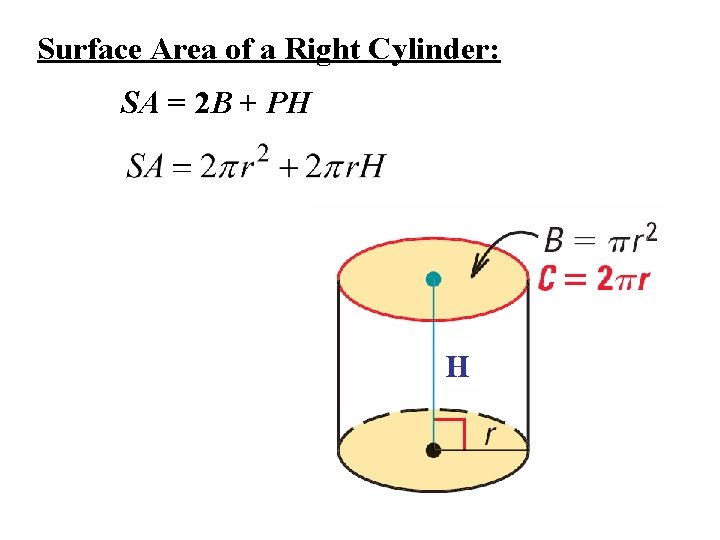Surface Area of a Right Cylinder: SA = 2 B + PH H1. Name the solid that can be formed by the net. Cylinder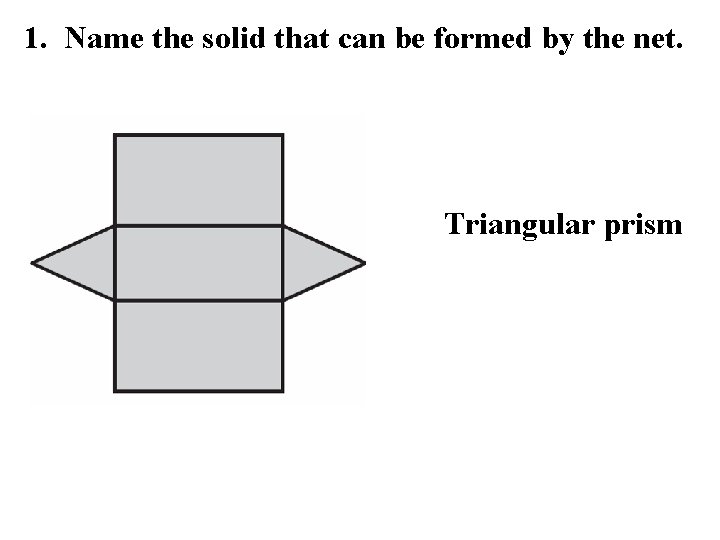1. Name the solid that can be formed by the net. Triangular prism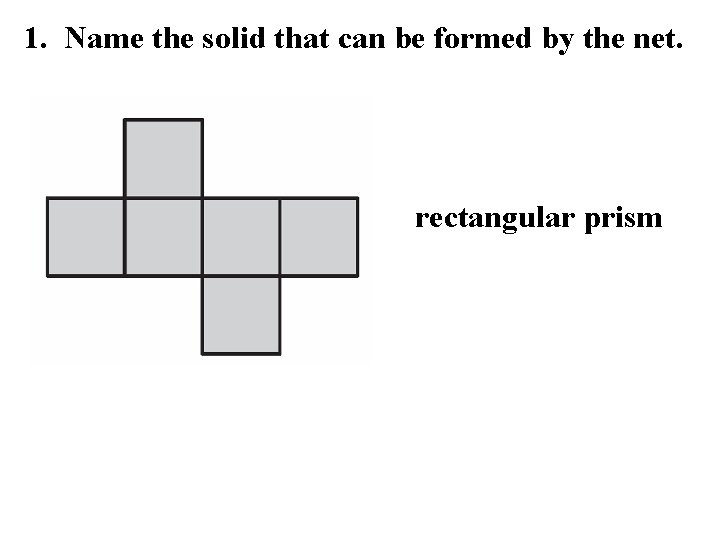1. Name the solid that can be formed by the net. rectangular prism2. Find the surface area of the right solid. SA = 2 B + PH SA = 2(30) + (22)(7) SA = 60 + 154 SA = 214 m 2 B = bh B = (5)(6) B = 30 P=5+6+5+6 P = 22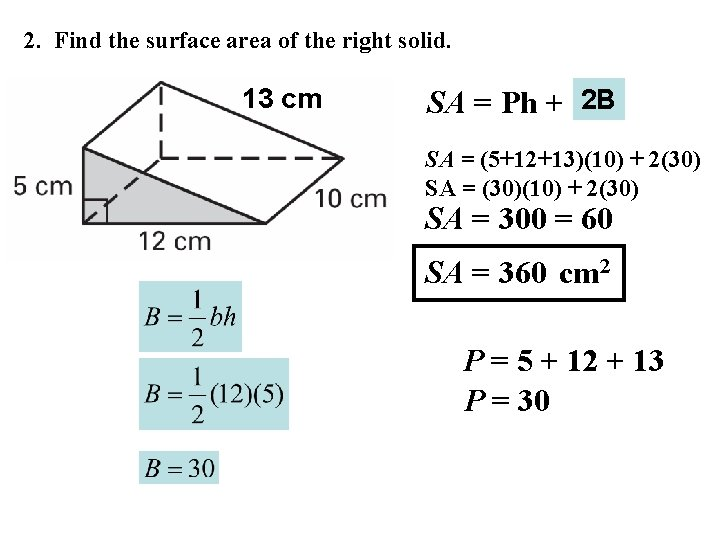2. Find the surface area of the right solid. 13 cm SA = Ph + 2 B SA = (5+12+13)(10) + 2(30) SA = (30)(10) + 2(30) SA = 300 = 60 SA = 360 cm 2 P = 5 + 12 + 13 P = 302. Find the surface area of the right solid. cm 2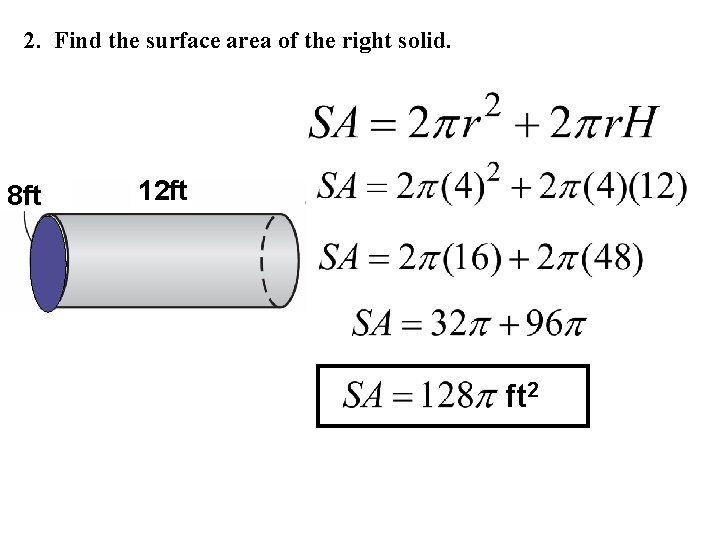2. Find the surface area of the right solid. 8 ft 12 ft ft 2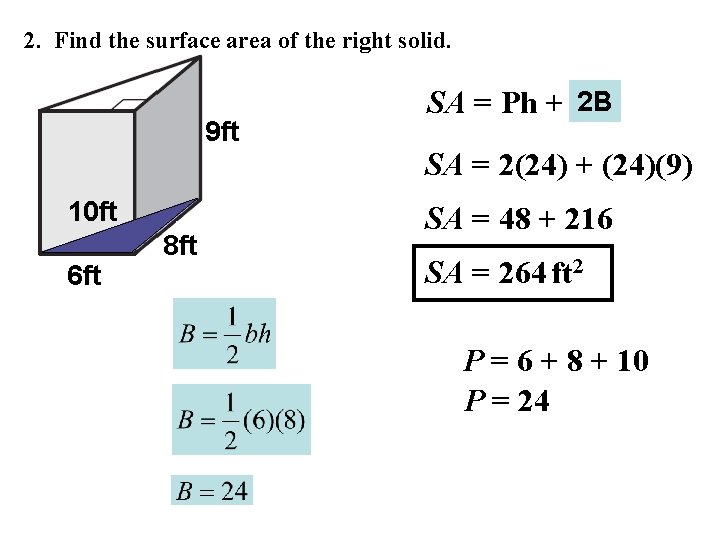2. Find the surface area of the right solid. 9 ft 10 ft 6 ft 8 ft SA = Ph + 2 B SA = 2(24) + (24)(9) SA = 48 + 216 SA = 264 ft 2 P = 6 + 8 + 10 P = 24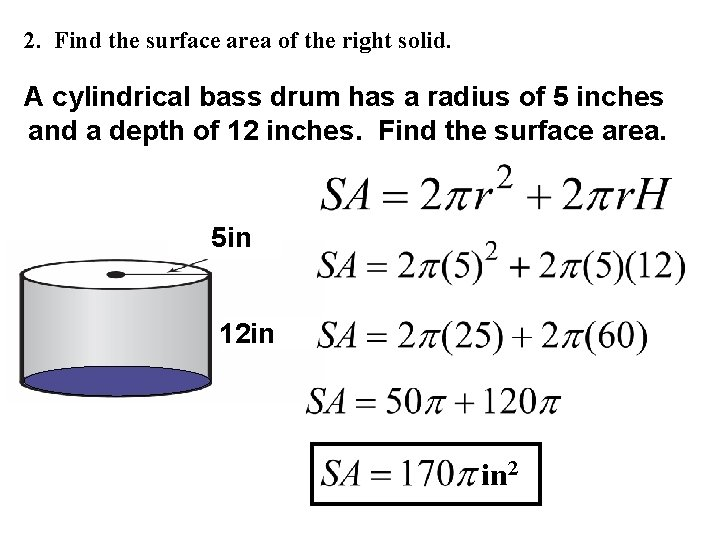2. Find the surface area of the right solid. A cylindrical bass drum has a radius of 5 inches and a depth of 12 inches. Find the surface area. 5 in 12 in in 2